Building AI: Neural Networks for beginners 👾

Teaching Machine to recognize Hand-written Numbers!

I am excited to share some of my experience studying machine learning with you, guys! I'm not an expert but I'll try to explain it the way I see it myself. I'm going to try to give you some intuition about how Neural Networks work, omitting most of the math to make it more understandable but, for the most curious of you, I'll leave the links to complete explanations/courses in the end.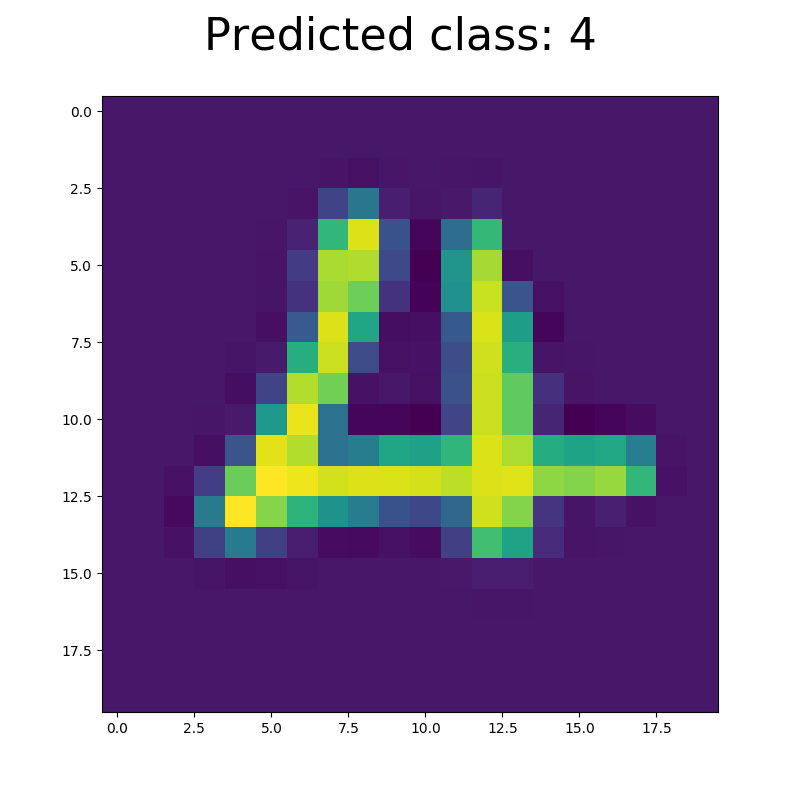In 29 mins, you'll be able to configure an algorithm that's going to recognize the written digits in python :)

## 🧠 What is a Neural Network?

Imagine Neural Network as an old wise wizard who knows everything and can predict your future by just looking at you.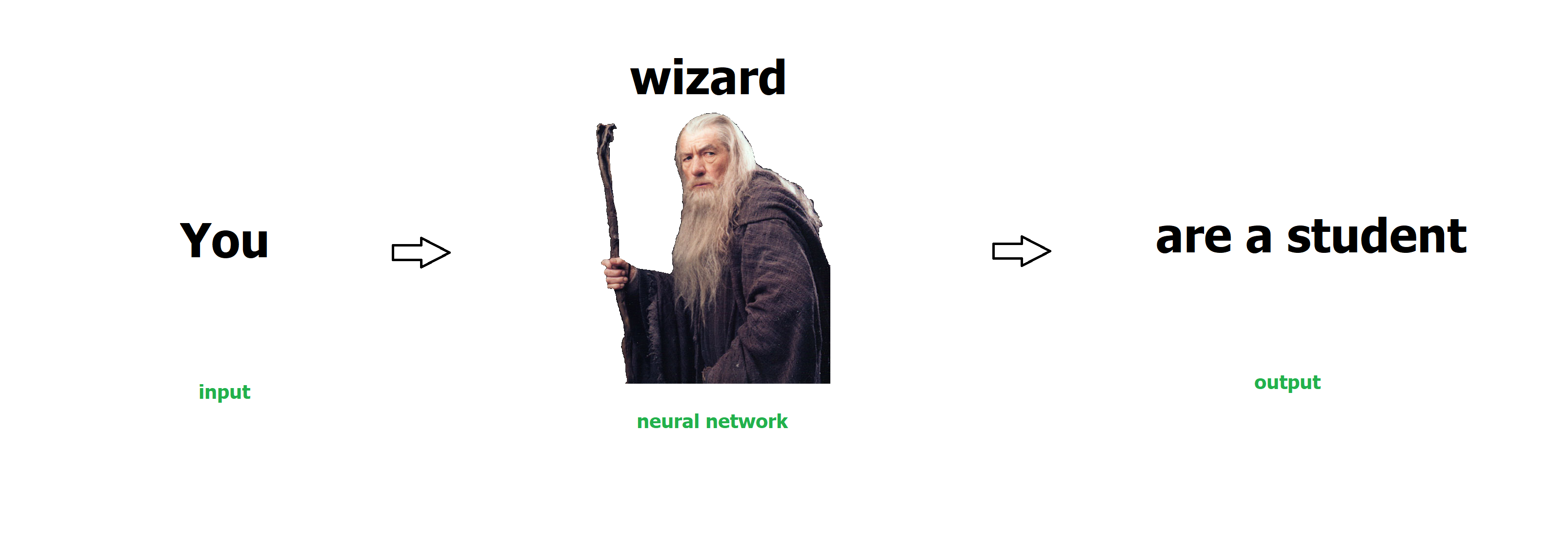It turns out that he manages to do so in a very non-magical way:

1. Before you visited him, he trained, carefully studied everything about many thousands of people who came to see him before you.

2. He now collects some data about what you look like (your apparent age, the website you found him at, etc).

3. He then compares it to the historical data he has about people that came to see him before.

4. Finally, he gives his best guess on what kind of person you are based on the similarities.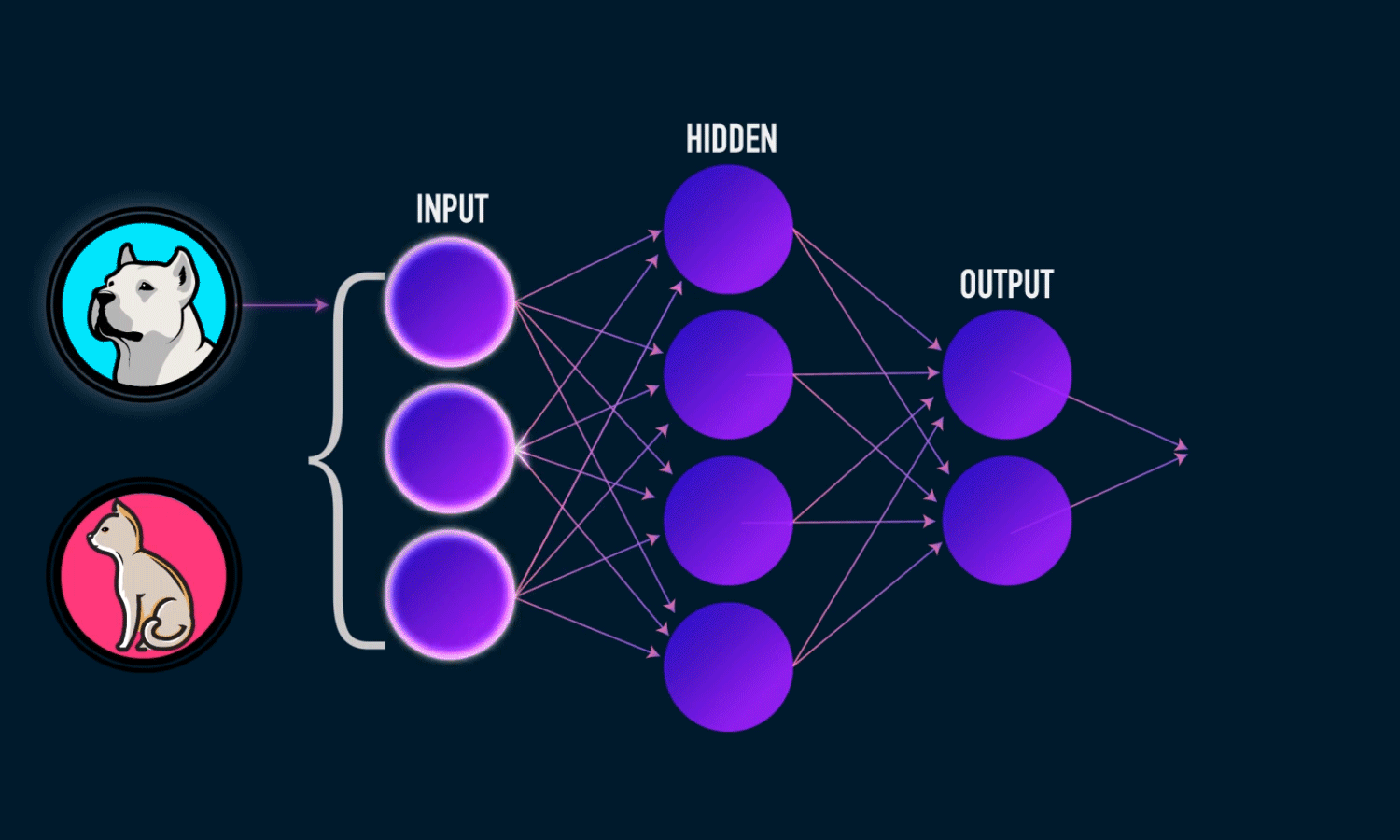In very general terms, it is the way many machine learning algorithms work. They are often used to predict things based on the history of similar situations: Amazon suggesting the product you might like to buy, or Gmail suggesting to finish the sentence for you, or a self-driving car learning to drive.

## 📙 Part 1: Import libraries

Let's start! I have put together a class that is doing all the math behind our algorithm and I'd gladly explain how it works in another tutorial or you could go through my comments and try to figure it out yourself if you know some machine learning.

For now, create a file called `NN.py` and paste this code:

``````import numpy as np
from scipy.optimize import minimize

class Neural_Network(object):

def configureNN(self, inputSize, hiddenSize, outputSize, W1 = np.array(), W2 = np.array(),
maxiter = 20, lambd = 0.1):
#parameters
self.inputSize = inputSize
self.outputSize = outputSize
self.hiddenSize = hiddenSize

#initialize weights / random by default
if(not W1.any()):
self.W1 = np.random.randn(
self.hiddenSize,
self.inputSize + 1)  # weight matrix from input to hidden layer
else: self.W1 = W1
if (not W2.any()):
self.W2 = np.random.randn(
self.outputSize,
self.hiddenSize + 1) # weight matrix from hidden to output layerself.W2 = W2
else: self.W2 = W2

# maximum number of iterations for optimization algorithm
self.maxiter = maxiter
# regularization penalty
self.lambd = lambd

#adds a column of ones to the beginning of an array
if (X.ndim == 1): return np.insert(X, 0, 1)
return np.concatenate((np.ones((len(X), 1)), X), axis=1)

def delBias(self, X):
#deletes a column from the beginning of an array
if (X.ndim == 1): return np.delete(X, 0)
return np.delete(X, 0, 1)

def unroll(self, X1, X2):
#unrolls two matrices into one vector
return np.concatenate((X1.reshape(X1.size), X2.reshape(X2.size)))

def sigmoid(self, s):
# activation function
return 1 / (1 + np.exp(-s))

def sigmoidPrime(self, s):
#derivative of sigmoid
return s * (1 - s)

def forward(self, X):
#forward propagation through our network
self.z = np.dot(
X,
self.W1.T)  # dot product of X (input) and first set of 3x2 weights
self.z2 = self.sigmoid(self.z)  # activation function
self.z3 = np.dot(
self.z2,
self.W2.T)  # dot product of hidden layer (z2) and second set of 3x1 weights
o = self.sigmoid(self.z3)  # final activation function
return o

def backward(self, X, y, o):
# backward propgate through the network
self.o_delta = o - y  # error in output

self.z2_error = self.o_delta.dot(
self.W2
)  # z2 error: how much our hidden layer weights contributed to output error
self.z2_delta = np.multiply(self.z2_error, self.sigmoidPrime(
self.z2))  # applying derivative of sigmoid to z2 error
self.z2_delta = self.delBias(self.z2_delta)

self.W1_delta += np.dot(
self.W2_delta += np.dot(
np.array([self.o_delta]).T, np.array([self.z2]))  # adjusting second set (hidden --> output) weights

def cost(self, nn_params, X, y):
#computing how well the function does. Less = better
self.W1_delta = 0
self.W2_delta = 0
m = len(X)

o = self.forward(X)
J = -1/m * sum(sum(y * np.log(o) + (1 - y) * np.log(1 - o))); #cost function
reg = (sum(sum(np.power(self.delBias(self.W1), 2))) + sum(
sum(np.power(self.delBias(self.W2), 2)))) * (self.lambd/(2*m)); #regularization: more precise
J = J + reg;

for i in range(m):
o = self.forward(X[i])
self.backward(X[i], y[i], o)
self.W1_delta = (1/m) * self.W1_delta + (self.lambd/m) * np.concatenate(
(np.zeros((len(self.W1),1)), self.delBias(self.W1)), axis=1)
self.W2_delta = (1/m) * self.W2_delta + (self.lambd/m) * np.concatenate(
(np.zeros((len(self.W2),1)), self.delBias(self.W2)), axis=1)

def train(self, X, y):
# using optimization algorithm to find best fit W1, W2
nn_params = self.unroll(self.W1, self.W2)
results = minimize(self.cost, x0=nn_params, args=(X, y),
options={'disp': True, 'maxiter':self.maxiter}, method="L-BFGS-B", jac=True)

self.W1 = np.reshape(results["x"][:self.hiddenSize * (self.inputSize + 1)],
(self.hiddenSize, self.inputSize + 1))

self.W2 = np.reshape(results["x"][self.hiddenSize * (self.inputSize + 1):],
(self.outputSize, self.hiddenSize + 1))

def saveWeights(self):
#sio.savemat('myWeights.mat', mdict={'W1': self.W1, 'W2' : self.W2})
np.savetxt('data/TrainedW1.in', self.W1, delimiter=',')
np.savetxt('data/TrainedW2.in', self.W2, delimiter=',')

def predict(self, X):
o = self.forward(X)
i = np.argmax(o)
o = o * 0
o[i] = 1
return o

def predictClass(self, X):
#printing out the number of the class, starting from 1
print("Predicted class out of", self.outputSize,"classes based on trained weights: ")
print("Input: \n" + str(X))
print("Class number: " + str(np.argmax( np.round(self.forward(X)) ) + 1))

def accuracy(self, X, y):
#printing out the accuracy
p = 0
m = len(X)
for i in range(m):
if (np.all(self.predict(X[i]) == y[i])): p += 1

print('Training Set Accuracy: {:.2f}%'.format(p * 100 / m))``````

## 📊 Part 2: Understanding Data

Cool! Now, much like the wizard who had to study all the other people who visited him before you, we need some data to study too. Before using any optimization algorithms, all the data scientists first try to understand the data they want to analyze.

Download files `X.in` (stores info about what people looked like - question) and `y.in`(stores info about what kind of people they were - answer) from here and put them into folder `data` in your repl.

• X: We are given 5,000 examples of 20x20 pixel pictures of handwritten digits from 0 to 9 (classes 1-10). Each picture's numerical representation is a single vector, which together with all the other examples forms an array `X`.
• Y: We also have an array `y`. Each column represents a corresponding example (one picture) from `X`. `y` has 10 rows for classes 1-10 and the value of only the correct class' row is one, the rest is zeros. It looks similar to this:
``````[0, 0, 0, 0, 0, 0, 0, 0, 0, 1] # represents digit 0 (class 10)
[1, 0, 0, 0, 0, 0, 0, 0, 0, 0] # represents digit 1 (class 1)
......
[1, 0, 0, 0, 0, 0, 0, 0, 1, 0] # represents digit 9 (class 9)``````

Now, let's plot it!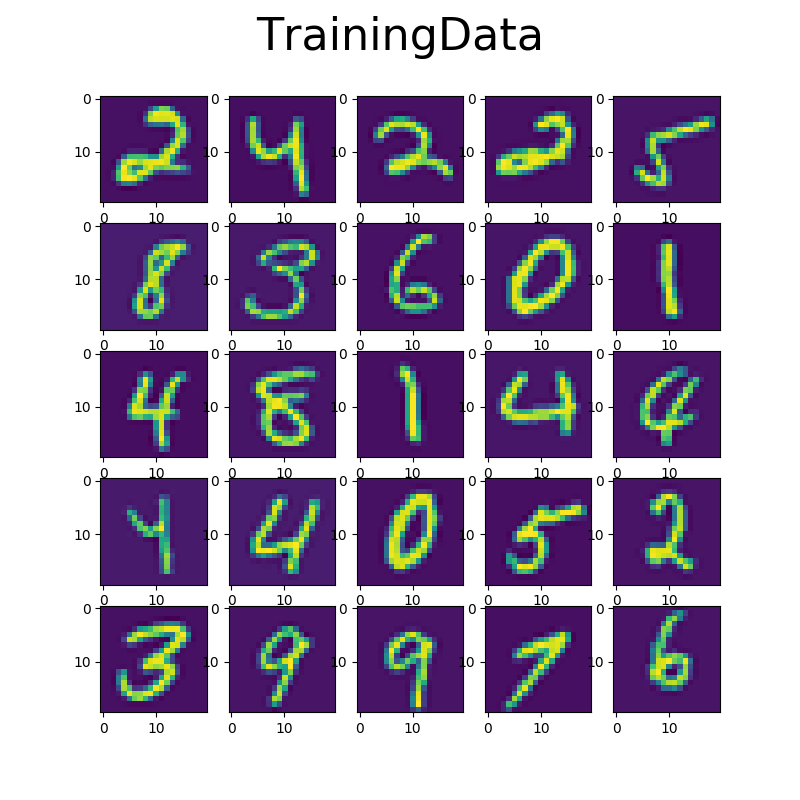In the end, I'd want a function `displayData(displaySize, data, selected, title)`, where

• `displaySize` - the numer of images shown in any one column or row of the figure,
• `data` - our X array,
• `selected` - an index (if displaying only one image) or vector of indices (if displaying multiple images) from X,
• `title` - the title of the figure

Create a `plots` folder to save your plots to. Also, if you use repl, create some empty file in the folder so that it doesn't disappear.

Create a `display.py` file and write the following code in there. Make sure to read the comments:

``````import matplotlib.pyplot as plt

# Displaying the data
def displayData( displaySize, data, selected, title ):

# setting up our plot
fig=plt.figure(figsize=(8, 8))
fig.suptitle(title, fontsize=32)
# configuring the number of images to display
columns = displaySize
rows = displaySize

for i in range(columns*rows):
# if we want to display multiple images,
# then 'selected' is a vector. Check if it is here:
if hasattr(selected, "__len__"): img = data[selected[i]]
else: img = data[selected]
img = img.reshape(20,20).transpose()
plt.imshow(img)

# We could also use plt.show(), but repl
# can't  display it. So let's insted save it
# into a file
plt.savefig('plots/' + title)

return None``````

Great, we are halfway there!

## 💪 Part 3: Training Neural Network

Now, after we understand what our data looks like, it's time to train on it. Let's make that wizard study!

It turns out that the results of the training process of the Neural Networks have to be stored in some values. These values are called parameters or weights of the Neural Network. If you were to start this project from scratch, your initial weights would be just some random numbers, however, it would take your computer forever to train to do such a complex task as recognizing digits. For this reason, I will provide you with the initial weights that are somewhat closer to the end result.

Download files `W1.in` and `W2.in` from here and put them into `data` folder.

We are now ready to write code to use our Neural Network library!Create a `train.py` file and write the following code in there. Make sure to read the comments:

``````# This code trains the Neural Network. In the end, you end up
# with best-fit parameters (weights W1 and W2) for the problem in folder 'data'
# and can use them to predict in predict.py
import numpy as np
import display
from NN import Neural_Network

NN = Neural_Network()

# Display inputs
sel = np.random.permutation(len(X));
sel = sel[0:100];
display.displayData(5, X, sel, 'TrainingData');

# Configuring settings of Neural Network:
#
# inputSize, hiddenSize, outputSize = number of elements
#                      in input, hidden, and output layers
# (optional) W1, W2  = random by default
# (optional) maxiter = number of iterations you allow the
#                      optimization algorithm.
#                      By default, set to 20
# (optional) lambd   = regularization penalty. By
#                      default, set to 0.1
#
NN.configureNN(400, 25, 10,
W1 = W1,
W2 = W2)

# Training Neural Network on our data
# This step takes 12 mins in Repl.it or 20 sec on your
# computer
NN.train(X, y)

# Saving Weights in the file
NN.saveWeights()

# Checking the accuracy of Neural Network
sel = np.random.permutation(5000)[1:1000]
NN.accuracy(X[sel], y[sel])``````

Now, you have to run this code either from:

• Repl.it - but you would need to move code from `train.py` into `main.py`. Don't delete `train.py` just yet. It would also take approximately 12 minutes to compute. You can watch this Crash Course video while waiting :)
• Your own computer - just run `train.py`, which takes 20 sec on my laptop to compute.

If you need help installing python, watch this tutorial.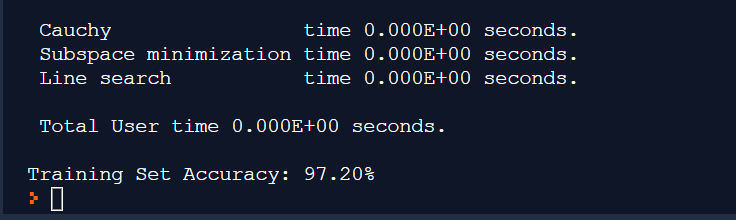## 🔮 Part 4: Predicting!

By now, you are supposed to have your new weights (`TrainedW1.in`,`TrainedW2.in`) saved in `data` folder and the accuracy of your Neural Network should be over 90%.

Let's now write a code to use the trained weights in order to predict the digits of any new image!Create a `predict.py` file and write the following code in there. Make sure to read the comments:

``````import numpy as np
import display
from NN import Neural_Network

NN = Neural_Network()

# Configuring settings of Neural Network:
NN.configureNN(400, 25, 10,
W1 = trW1,
W2 = trW2)

# Predicting a class number of given input
testNo = 3402; # any number between 0 and 4999 to test
NN.predictClass(X[testNo])
# Display output
display.displayData(1, X, testNo, 'Predicted class: ' + str(np.argmax(np.round(NN.forward(X[testNo]))) + 1) )``````

Change the value of `testNo` to any number between 0 and 4999. In order to get a digit (class) prediction on the corresponding example from array X, run the code from:

• Repl.it - but you would need to move code from `predict.py` into `main.py`. Don't delete `predict.py` just yet.
• Your own computer - just run `predict.py`.

Yay, you are officially a data scientist! You have successfully:

1. Analyzed the data

2. Implemented the training of your Neural Network

3. Developed a code to predict new testing examples## 🚀 Acknowledgments

Hat tip to @shamdasani whose code I used as a template for Neural Network architecture and Andrew Ng from Stanford whose data I used.

Plenty of things I told you are not completely correct because I rather tried to get you excited about the topic I am passionate about, not dump some math on you!

If you guys seem to enjoy it, please follow through with studying machine learning because it is just an amazing experience. I encourage you to take this free online course on it to learn the true way it works.

Also, it's my first post here and I'd appreciate any feedback on it to get better.

Keep me updated on your progress, ask any questions, and stay excited! ✨✨✨

You are viewing a single comment. View All
ArtemLaptiev1 (89)

@21Miya I actually did not know that. Thanks for pointing it out :D